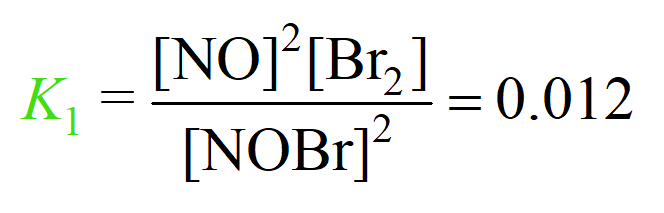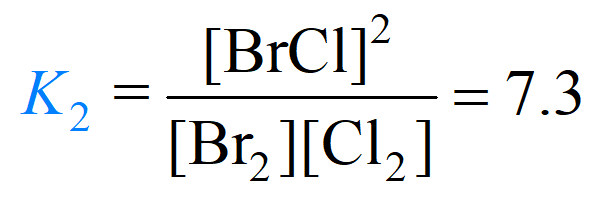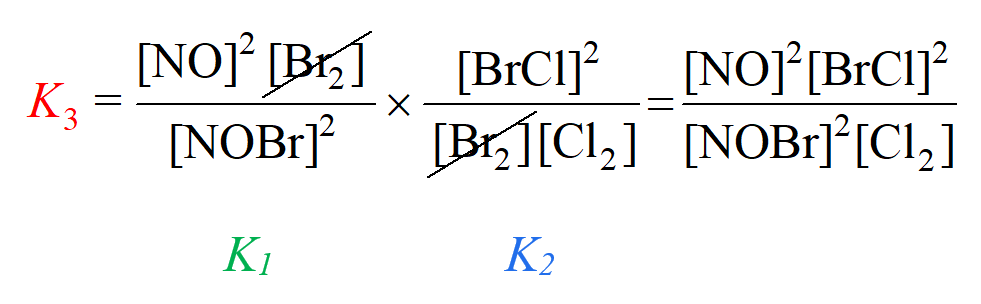## General Chemistry

Some reactions can be represented as a sequence of two or more relations. For example, let’s consider the following reaction between NOBr and Cl2 gases:

2NOBr(g) + Cl2(g) ⇆ 2NO(g) + 2 BrCl(g)

We can represent this net transformation as a sum of two reactions:

1) 2NOBr(g) ⇆ 2NO(g) + Br2(g)

2) Br2(g) + Cl2(g) ⇆ 2BrCl(g)

To confirm that these two give the target equation, simply add them up and cancel out the identical components on both sides of the equation:

1) 2NOBr(g) ⇆ 2NO(g) + Br2(g)
+
2) Br2(g) + Cl2(g) ⇆ 2BrCl(g)

2NOBr(g) + Br2(g) + Cl2(g) ⇆ 2NO(g) + Br2(g) + 2 BrCl(g)

_______________________________________________

3) 2NOBr(g) + Cl2(g) ⇆ 2NO(g) + 2 BrCl(g)

So, Br2 appeared on both sides of the equation and by canceling it, we obtained the final equation (3).

Now, how is this related to the equilibrium constant?

It turns out that we can calculate the equilibrium constant for a reaction if we know the equilibrium constants for other reactions that add up to this reaction. For this, you simply need to multiply the equilibrium constants of the two reactions.

For example, suppose the equilibrium constants of reactions (1) and (2) are 0.012 and 7.2 respectively:

1) 2NOBr(g) ⇆ 2NO(g) + Br2(g)2) Br2(g) + Cl2(g) ⇆ 2BrCl(g)To obtain the equilibrium constant for reaction (3), we multiply the ones for reaction (1) and (2):

2NOBr(g) + Cl2(g) ⇆ 2NO(g) + 2BrCl(g) (3)Notice that we got an expression for K3 that matches what we’d write according to the chemical equation (3).

Therefore, the value of K3 is the product of K1 and K2:

K3 = K1 x K2 = 0.012 x 7.3 = 0.088

If this is confusing, you can review the Hess’s law which uses a similar strategy to calculate the enthalpy of a reaction based on the enthalpy of other reactions that add up to it.

#### Practice

1.

The equilibrium constant values for the reactions below were determined at a certain temperature:

S(s) + O2(g) ⇆ SO2(g)    Ka = 3.2 x 1045

2S(s) + 3O2(g) ⇆ 2SO3(g)    Kb = 3.2 x 10124

Using these data, determine the equilibrium constant Kc for the following reaction:

2SO2(g) + O2(g) ⇆ 2SO3(g)    Kc = ?

This content is available to registered users only. Click here to Register! By joining Chemistry Steps, you will gain instant access to the answers and solutions for all the Practice Problems and the powerful set of General Chemistry 1 and 2 Summary Study Guides.
Solution
This content is available to registered users only. Click here to Register! By joining Chemistry Steps, you will gain instant access to the answers and solutions for all the Practice Problems and the powerful set of General Chemistry 1 and 2 Summary Study Guides.
2.

The equilibrium constant values for the reactions below were determined at a certain temperature:

2NO(g) ⇆ N2(g) + O2(g) Ka = 3.8 x 1032

NO(g) + 1/2Cl2(g) ⇆ NOCl(gKb = 6.4

Using these data, determine the equilibrium constant Kc for the following reaction:

1/2N2(g) + 1/2O2(g) + 1/2Cl2(g) ⇆ NOCl(gKc = ?